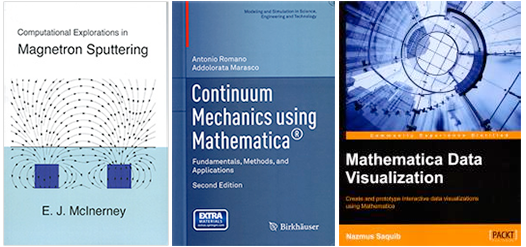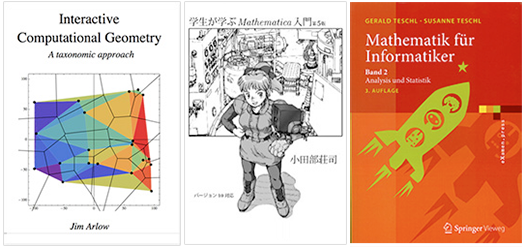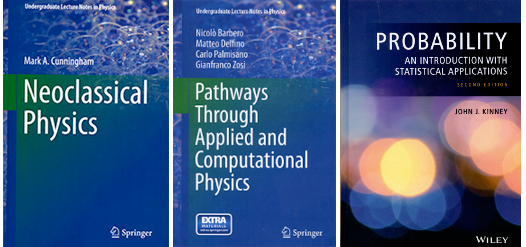Browse by Topic

# New Wolfram Technologies Books

We are once again thrilled by the wide variety of topics covered by authors around the world using Wolfram technologies to write their books and explore their disciplines. These latest additions range from covering the basics for students to working within specialties like continuum mechanics.Computational Explorations in Magnetron Sputtering
Magnetron sputtering is a widely used industrial process for depositing thin films. E. J. McInerney walks you through the physics of magnetron sputtering in a step-by-step fashion using Mathematica syntax and functions. The reader is encouraged to explore this fascinating topic by actively following along with a series of simple computer models. By working through these models, readers can build their intuition of PVD processes and develop a deeper appreciation of the underlying physics.

Continuum Mechanics using Mathematica: Fundamentals, Methods, and Applications, second edition
In this second edition of Continuum Mechanics using Mathematica, Antonio Romano and Addolorata Marasco take a methodological approach that familiarizes readers with the mathematical tools required to correctly define and solve problems in continuum mechanics. It provides a solid basis for a deeper study of more challenging and specialized problems related to nonlinear elasticity, polar continua, mixtures, piezoelectricity, ferroelectricity, magneto-fluid mechanics, and state changes.

Mathematica Data Visualization: Create and Prototype Interactive Data Visualizations Using Mathematica
Nazmus Saquib begins by introducing you to the Mathematica environment and the basics of dataset loading and cleaning. You will then learn about different kinds of widely used datasets, time series, and scientific, statistical, information, and map visualizations. Each topic is demonstrated by walking you through an example project. Along the way, the dynamic interactivity and graphics packages are also introduced. This book teaches you how to build visualizations from scratch, quickly and efficiently.Interactive Computational Geometry, A Taxonomic Approach
This ebook by Jim Arlow is an interactive introduction to some of the fundamental algorithms of computational geometry. The code base, which is in the Wolfram Language, is integrated into the text and is fully executable. This book is delivered as an interactive CDF (Computable Document Format) file that is viewable in the free CDF Player available from Wolfram, or can be opened in Mathematica. Readers are encouraged to have a copy of Mathematica in order to get the most out of this text.

Souji Otabe provides a quick introduction to Mathematica for students. Engineers and scientists need to know about symbolic computation, but they also need to know numeric computation to analyze experimental data. In this ebook, Mathematica is treated as a general problem-solving tool for science and engineering students. Readers will study basic operations of Mathematica first, and then they will learn how Mathematica can be applied to engineering and science.

Mathematik fur Informatiker (Mathematics for Computer Scientists) Band 2: Analysis und Statistik 3. Auflage
The second edition of Gerald and Susanne Teschl’s textbook introduces mathematical foundations that are concise, yet vivid and easy to follow. They are illustrated using numerous worked-out examples mixed with applications to computer science, historic background, and connections to related areas. The end of every chapter offers a self-test and warmup problems (with detailed solutions), as well as more advanced problems. Complementary sections introduce Mathematica to visualize the subjects taught, thereby fostering comprehension of the material.Neoclassical Physics
In this introductory text by Mark A. Cunningham, physics concepts are introduced as a means of understanding experimental observations, not as a sequential list of facts to be memorized. Numerous exercises are provided that utilize Mathematica software to help students explore how the language of mathematics is used to describe physical phenomena. Students will obtain much more detailed information about covered topics and will also gain proficiency with Mathematica, a powerful tool with many potential uses in subsequent courses.

Pathways Through Applied and Computational Physics
This book is the collaborative effort of Nicolo Barbero, Matteo Delfino, Carlo Palmisano, and Gianfranco Zosi to illustrate the role that different branches of physics and mathematics play in the execution of actual experiments. The unique feature of the book is that all the subjects addressed are strictly interconnected within the context of the execution of a single experiment with very high accuracy, namely the redetermination of the Avogadro constant NA, one of the fundamental physical constants. Another essential feature is the focus on the role of Mathematica, an invaluable, fully integrated software environment for handling diverse scientific and technical computations.

Probability: An Introduction with Statistical Applications, second edition
Thoroughly updated, John J. Kinney’s second edition of this title features a comprehensive exploration of statistical data analysis as an application of probability. The new edition provides an introduction to statistics with accessible coverage of reliability, acceptance sampling, confidence intervals, hypothesis testing, and simple linear regression. Encouraging readers to develop a deeper intuitive understanding of probability, the author presents illustrative geometrical presentations and arguments without the need for rigorous mathematical proofs. This book features an appendix dedicated to the use of Mathematica and a companion website containing the referenced data sets.